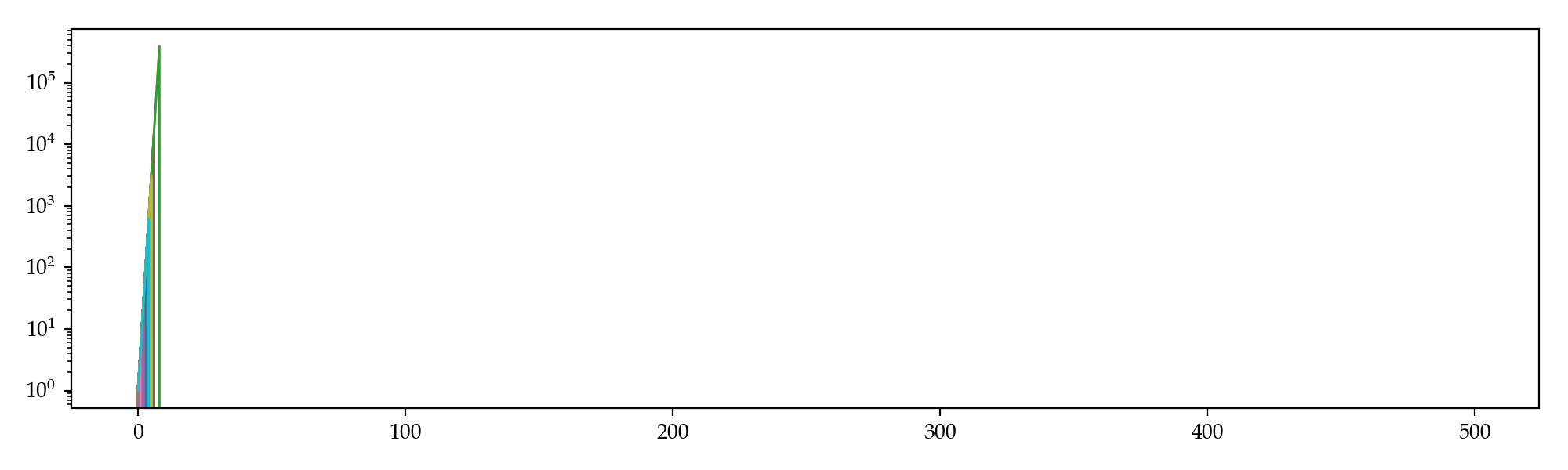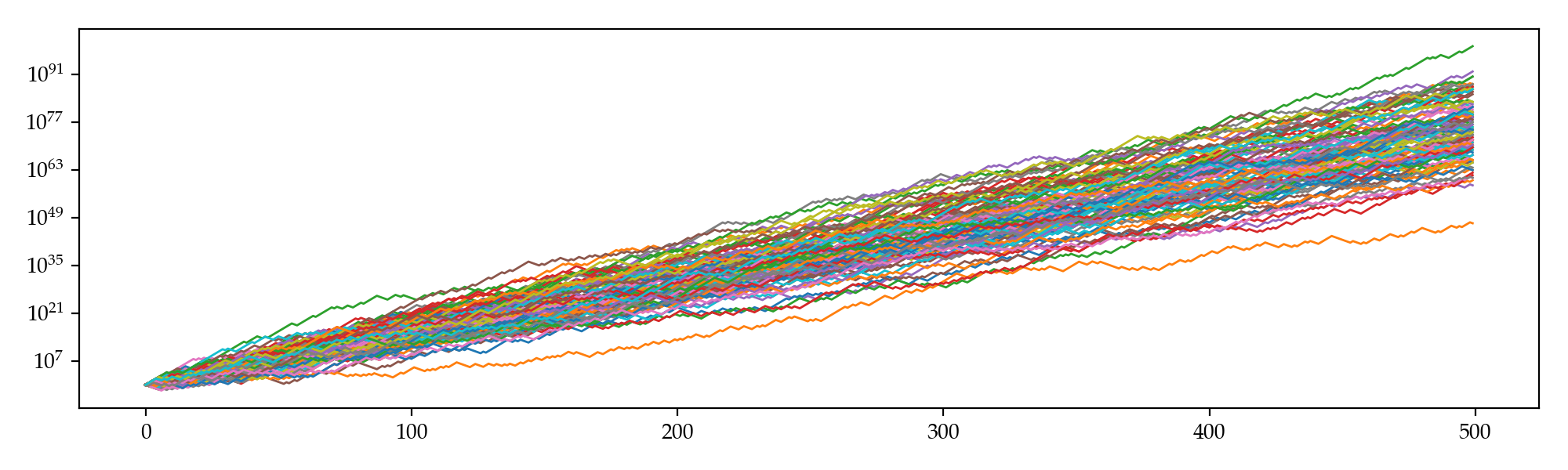I am recently playing a billiard game where you can play a series of exciting tournaments. In each tournament, you pay an entrance fee of, for example, $\500$, to potentially win a prize of, say, $\2500$. There are various kinds of tournaments with different entrance fees ranging from $\100$ up to over $\10000$. After hundreds of games, my winning rate stablized around $58\%$, which is actually pretty good as it significantly beats random draws. A natural concept therefore came into my mind: Is there an optimal strategy?

Well, I think so. I'll list two strategies below and try to explore any potential optimality. We can reasonably model these tournaments as repetitive betting with certain fixed physical probability $p$ of winning and odds of $(d-1)$:$1$ against ourselves. Given that there are sufficiently sparse tournament entrance fees, we may model these fees as a real variable $x\in\mathbb{R}_+$ to maximize our long run profitability. Without loss of generality, let's assume an initial balance of $M_0=0$ and that money in this world is infinitely divisible. The problem then becomes determination of the optimal $x\in[0,1]$ s.t. the expected return is maximized. Nonetheless, regarding different interpretations of this problem we have several solutions. Some are intriguing while others may be frustrating.

# Martingale

Let's first take a look at potential values of $x$ and the corresponding balance trajectories $M_t$. For any $0 \le x \le 1$, we have probability $p$ to get an $x$-fraction of our whole balance $D$-folded and $1-p$ to lose it, that is $\text{E}(M_{t+1}\mid\mathcal{F}_t) = (1-x)M_t + p\cdot xdM_t + (1-p)\cdot 0 = [1 + (pd-1)x] M_t$ which indicates $M_t$ is a sub-martingale as in this particular case, $p=0.58$, $d=5$ and thus $pd=2.9 > 1$. So the optimal fraction is $x^* = 1$, which is rather aggresive and yields a ruin probability of $1-p^n$ for the first $n$ bets. Simulation supports our worries: not once did we survived $10$ bets in this tournament, and the maximum we ever attained is less than a million.# Kelly Criterion

If consider $\log M_t$ instead, then \begin{align*} \text{E}(\log M_t\mid \mathcal{F}_t) &= p\cdot \log[(1-x)M_t + xdM_t] + (1-p)\cdot \log[(1-x)M_t + 0]\\ &= p\cdot \log[(1-(1-d)x)M_t] + (1-p)\cdot \log[(1-x)M_t]. \end{align*} The first order condition is $-\frac{\partial}{\partial x}\text{E}(\log M_t\mid \mathcal{F}_t) = \frac{p(1-d)}{1-(1-d)x}+\frac{1-p}{1-x} = 0 \quad\Rightarrow\quad x^* = \frac{pd-1}{d-1}=0.475$ which is more conservative and therefore, should survive longer than the previous strategy. Simulation gives the following trajectories: even the worst sim beat the best we got when $x=1$.# Further Thoughts

According to Doob's martingale inequality, the probability of our balance ever attaining a value no less than $C = 1\times10^{60}$ in $T=500$ steps is $\text{P}\left(\sup_{t \le T}M_t\ge C\right) \le \frac{\text{E}(M_T)}{C} = \frac{M_0}{C} \prod_{t=0}^{T-1}\frac{\text{E}(M_{t+1}\mid\mathcal{F}_t)}{M_t} = \frac{[1+(pd-1)x]^T}{C} \approx 4.6\times10^{139} \gg 1.$ This implies the superior limit of the probability that our balance exceeds $1\times10^{60}$ within $500$ steps is one (instead of what simulation gave us, which is around $0.31$). To put it differently, we actually might be able to find a certain strategy that is even significantly better than the one given by the Kelly criterion.

What is it, then? Or, does it actually exist? I don't have an idea yet, but perhaps exploratory algorithms like machine learning will give us some hints, and perhaps the strategy is not static but rather dynamic.

1. 1.In a game with odds $(d-1)$:$1$, for each dollar you bet, you either win $d$ dollars or lose all. ↩︎
2. 2.$M_t$ is a sub-martingale iff. $\text{E}(M_{t+1}\mid \mathcal{F}_t) \ge M_t$ for all $t \ge 0$. ↩︎
3. 3.Let $X_t$ be a non-negative sub-martingale, then $\text{P}(\sup_{t\le T} X_t \ge C)\le \text{E}(X_T) / C$. ↩︎